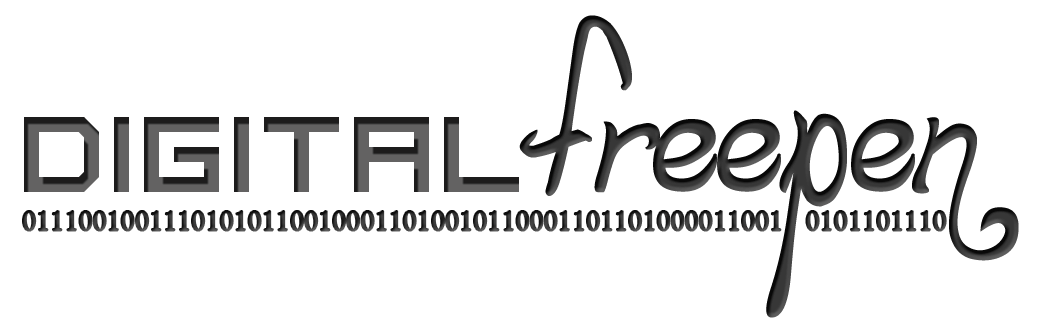Oct 1 2012

Our introductory computer science class at Waterloo uses a functional programming language called Scheme. However, when it comes to explaining concepts, our professor uses Haskell, though we are not required to actually learn how to program with it (something to do with cryptic compiler errors). Why so?

So at 11:30 yesterday night, being bored, I tried writing some code in Haskell. Below is an implementation of mergesort (and probably not the most efficient one, but the best I could do not knowing any prebuilt Haskell functions).

-- count the number of elements in a list
count [] = 0
count (y:ys) = 1 + (count ys)

-- get the first n elements of a list
getFirstN n (y:ys) | (n == 0)   = []
| otherwise  = y : (getFirstN (n - 1) ys)

-- get the last n elements of a list
getLastNHelp n2 (y:ys) | (n2 == 1)    = ys
| otherwise    = getLastNHelp (n2 - 1) ys
getLastN n lst = getLastNHelp ((count lst) - n) lst

-- merge two sorted lists as to have the output list sorted also
merge [] lst = lst
merge lst [] = lst
merge (x:xs) (y:ys) | (x < y)   = x : (merge xs (y:ys))
| otherwise = y : (merge (x:xs) ys)

-- sort a list using mergesort
mergesort [] = []
mergesort [x] = [x]
mergesort lst = merge (mergesort (getFirstN (floor ((count lst) / 2)) lst))
(mergesort (getLastN (ceiling ((count lst) / 2)) lst))


Isn't so neat and so short? Every line is relevant piece of code that accomplishes something other than moving pointers around. It also took only 40 minutes to learn the language and write this. Doing the same for something like C++ seems like it would be quite a challenge even for experienced programmers.

I'll have to find stuff to do with Haskell since it's definitely worth pushing further.